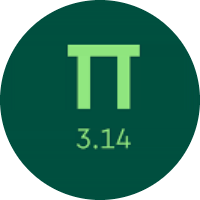# Learning the Multiplication and Division of Fractions

In this quiz, you will learn about the multiplication and division of fractions. You will find problems on different types of multiplications and the division of fractions.

Start Quiz

Multiply.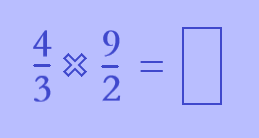3/2

6

3

Which sign makes the statement true?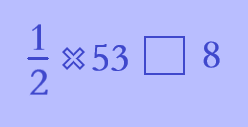<

=

>

Multiply.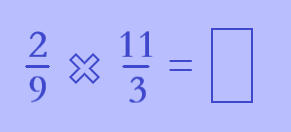24/27

24/9

22/27

Evaluate.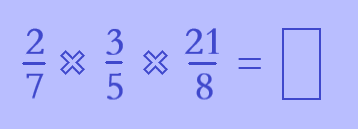18/20

9/20

42/40

Multiply.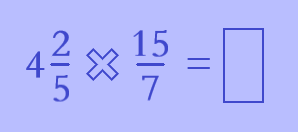66/7

56/35

What is the reciprocal of 10/9?

9/10

5/3

Divide.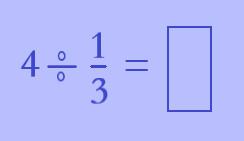4/3

3/4

12

Divide.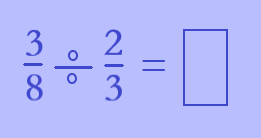6/24

9/16

3/16

Which sign makes the statement true?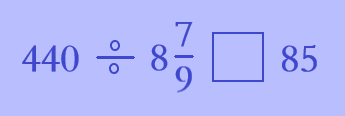<

=

>

Evaluate.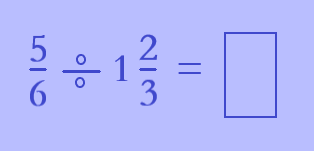1/2

3/5

2/3

Quiz/Test Summary
Title: Learning the Multiplication and Division of Fractions
Questions: 10
Contributed by: